JEE  >  WBJEE Subjective Phy & Chem Sample Paper

# WBJEE Subjective Phy & Chem Sample Paper Notes | Study WBJEE Sample Papers, Section Wise & Full Mock Tests - JEE

## Document Description: WBJEE Subjective Phy & Chem Sample Paper for JEE 2022 is part of Sample Paper for WBJEE Sample Papers, Section Wise & Full Mock Tests preparation. The notes and questions for WBJEE Subjective Phy & Chem Sample Paper have been prepared according to the JEE exam syllabus. Information about WBJEE Subjective Phy & Chem Sample Paper covers topics like and WBJEE Subjective Phy & Chem Sample Paper Example, for JEE 2022 Exam. Find important definitions, questions, notes, meanings, examples, exercises and tests below for WBJEE Subjective Phy & Chem Sample Paper.

Introduction of WBJEE Subjective Phy & Chem Sample Paper in English is available as part of our WBJEE Sample Papers, Section Wise & Full Mock Tests for JEE & WBJEE Subjective Phy & Chem Sample Paper in Hindi for WBJEE Sample Papers, Section Wise & Full Mock Tests course. Download more important topics related with Sample Paper, notes, lectures and mock test series for JEE Exam by signing up for free. JEE: WBJEE Subjective Phy & Chem Sample Paper Notes | Study WBJEE Sample Papers, Section Wise & Full Mock Tests - JEE
 1 Crore+ students have signed up on EduRev. Have you?

Question: 1. The displacement x of a particle at time t moving under a constant force is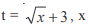in meters, t in seconds. Find the work done by the force in the interval from t = 0 to t = 6 second.
Ans: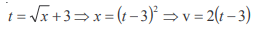v at t = 0, – 6 m/s
v at t = 6 sec., 6 m/s
change in KE is zero ⇒ work done = 0

Question: 2. Calculate the distance above and below the surface of the earth at which the acceleration due to gravity is the same
Ans:on solving we get
– Rh + R2 – h2 = 0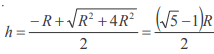Question: 3. A ray of light travelling inside a rectangular glass block of refractive index √2 is incident on the glass-air surface at an angle of incidence of 45°. Show that the ray will emerge into the air at an angle of refraction equal to 90°
Ans:  Given C = 45°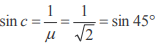So the ray will graze the interface after refraction at an angle of 90°

Question: 4. Two cells each of same e.m.f ‘e’ but of internal resistances r1 and r2 are connected in series through an external resistance R. If the potential difference between the ends of the first cell is zero, what will be the value of R in terms r1 and r2 ?
Ans: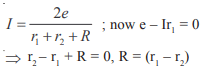Question: 5. At time t = 0, a radioactive sample has a mass of 10 gm. Calculate the expected mass of radioactive sample after two successive mean lives.
Ans:
Two successive mean lives =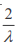No. of nuclei after two mean lives =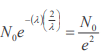Therefore mass =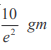Question: 6. Calculate the number of H+ ion present in 1 ml of a solution whose pH is 10.
Ans: pH = 10
[H+] = 10–10 M
In 1000 ml solution there are 6.023 × 1013 H+ ions
In 1 ml solution there are 6.023 × 1010 H+ ions

Question: 7.
Give the structure of pyro-sulfuric acid. How would you prepare it? What would you observe when colourless HI is added to pyro-sulfuric acid?
Ans: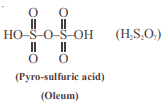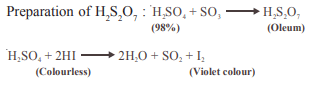Question: 8. Write with a balanced chemical equation how gypsum is used for the conversion of ammonia into ammonium sulfate without using H2 SO4 .
Ans:  Balanced reaction is
2NH3 + CaSO4 + CO2 + H2O = (NH4 )2 SO4 + CaCO3

Question: 9.  Convert phenol to p-hydroxy acetophenone in not more than 2 steps.
Ans: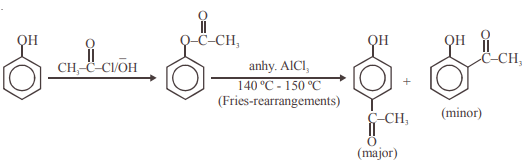Question: 10. An organic compound ‘A’ on treatment with ammoniacal silver nitrate gives metallic silver and produces a yellow crystalline precipitate of molecular formula C9H10N4O4 , on treatment with Brady’s reagent. Give the structure of the organic compound ‘A’.
Ans:  Compound (A) is an aldehyde. It should be propanal CH3CH2CHO
Reactions :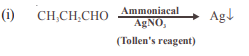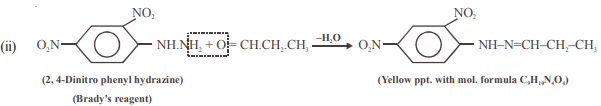The document WBJEE Subjective Phy & Chem Sample Paper Notes | Study WBJEE Sample Papers, Section Wise & Full Mock Tests - JEE is a part of the JEE Course WBJEE Sample Papers, Section Wise & Full Mock Tests.
All you need of JEE at this link: JEE

## WBJEE Sample Papers, Section Wise & Full Mock Tests

4 videos|10 docs|54 tests
 Use Code STAYHOME200 and get INR 200 additional OFF

## WBJEE Sample Papers, Section Wise & Full Mock Tests

4 videos|10 docs|54 tests

Track your progress, build streaks, highlight & save important lessons and more!

,

,

,

,

,

,

,

,

,

,

,

,

,

,

,

,

,

,

,

,

,

,

,

,

;